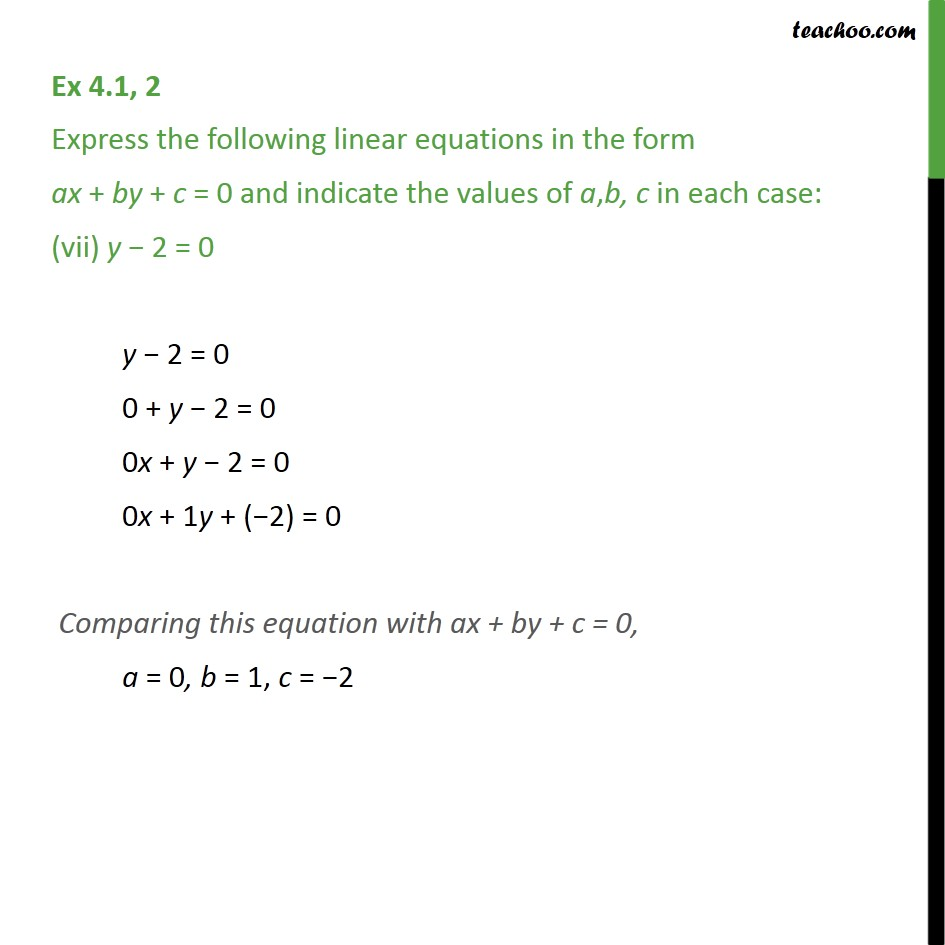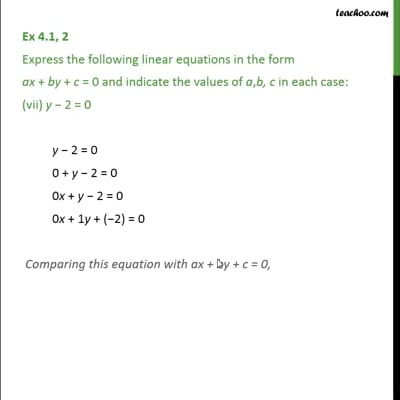Ex 4.1

Chapter 4 Class 9 Linear Equations in Two Variables
Serial order wiseThis video is only available for Teachoo black users

Maths Crash Course - Live lectures + all videos + Real time Doubt solving!

### Transcript

Ex 4.1, 2 Express the following linear equations in the form ax + by + c = 0 and indicate the values of a,b, c in each case: (vii) y − 2 = 0 y − 2 = 0 0 + y − 2 = 0 0x + y − 2 = 0 0x + 1y + (−2) = 0 Comparing this equation with ax + by + c = 0, a = 0, b = 1, c = −2